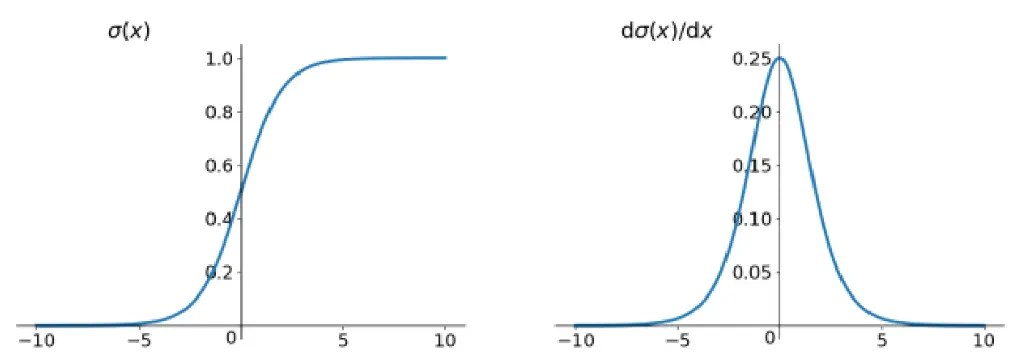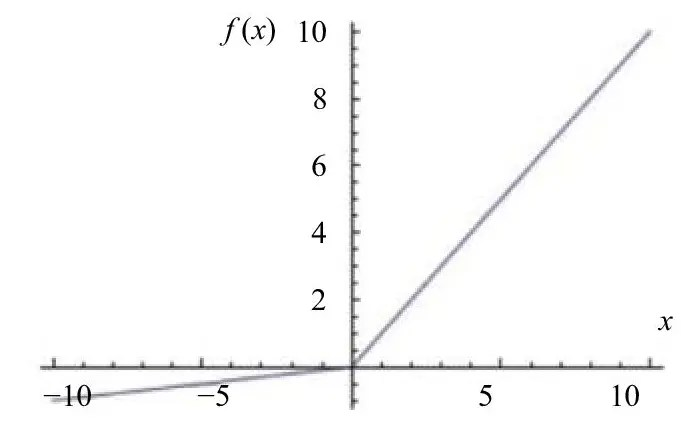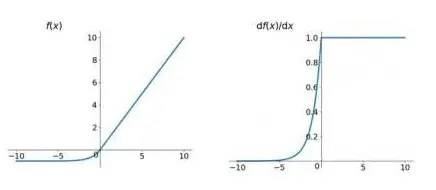# Activation function

Posted May 27, 20205 min read

# introduction

In the process of learning the neural network, you will always hear the activation function(excitation function). The activation function is the source of nonlinearity in the neural network. If these activation functions are removed, the neural network only has linear operations, and the final effect is quite For a single-layer linear model, this article mainly explains the activation function from the following aspects:

• What is the activation function
• Classification and characteristics of activation function
• How to choose the right activation function

# What is the activation function

First of all, we must first understand the basic model of neural network(unfamiliar can go to another article in this article: introduction to neural network ) The single neural network model is shown in the figure:SUM represents the neuron, xi represents the input layer, wi represents the weight, t represents the output layer, the input is multiplied by the corresponding weight input to the neuron, and passed to the output layer, there is a functional relationship f between the input and output, This function is called the activation function(excitation function).

# Classification and characteristics of activation functions

## Sigmoid function

Sigmoid is a commonly used nonlinear activation function, the mathematical form is as follows:

\$\$f(z) = \ frac {1} {1+ e ^ {-z}} \$\$

The geometric image and its derivatives are shown in the figure:Features:

1. The output range is limited \ [0,1 ], the data is not easy to diverge during the transmission process
2. The probability value can be expressed in the output layer.

1. The output is not zero-centered
2. The gradient descent is very obvious, and the two ends are too flat, and the gradient disappears easily. This is because the Sigmoid derivative is less than 0.25. During back propagation, the gradient multiplication will slowly approach 0, and the output value The domain is asymmetric.
3. Mathematical expressions contain power operations, which will greatly increase the training time for large-scale deep networks.
4. The left end tends to 0, the right end tends to 1, and both ends tend to saturate.

## Hyperbolic tangent function(tanh)

The mathematical form is as follows:

\$\$tanh(x) = \ frac {e ^ {x} -e ^ {-x}} {e ^ {x} + e ^ {-x}} \$\$

The tanh(x) function and its derivatives are shown below:Features:

1. Map the data to \ [-1,1 ], which solves the problem of asymmetry in the output value range of the Sigmoid function.
2. Differentiable and antisymmetric, the center of symmetry is at the origin, and the output is zero-centered.

1. The output value field is still flat at both ends, and the problem of gradient disappearance still exists.
2. Still exponential calculation
3. Both ends tend to be saturated

## ReLU function

The ReLu function is a commonly used activation function in neural networks. The mathematical form is as follows:

\$\$f(x) = relu(x) = max(x, 0) \$\$

The geometric image and its derivatives are shown below:The picture on the left is the ReLU function image. As you can see, the value of the negative semi-axis is 0, and the positive half-axis is a linear function.
Features:

1. The positive semi-axis is linear, so there is no gradient saturation phenomenon in the positive semi-axis;
2. The convergence speed is faster than Sigmoid and Tanh;
3. The calculation is more efficient and only requires a threshold to get the activation value.

1. The output of ReLU is not zero-centered;
2. Dead ReLU Problem means "death neuron", which means that certain neurons will never be activated, resulting in the corresponding parameters cannot be updated. There are two main reasons for this situation:(1) Parameter initialization, which is relatively rare.(2) If the learning rate is too high, the parameter update during the training process is too large. Unfortunately, the network enters this state. You can use the Xavier initialization method to solve, or use adagrad and other algorithms to automatically adjust the learning rate.

to sum up
Although ReLU has these two problems, it is still the most commonly used activation function at present, because its non-linearity is relatively weak, and the neural network is generally deeper, because the deeper network has better generalization ability.

## Linear neuron with leak correction(Leaky ReLU function(PReLU))

The mathematical expression is:

\$\$f(x) = \ \ {{\ alpha x, \ x <0 \ \ atop x, \ x \ geq0} \$\$

The geometric image is shown below:Features:

1. To solve the Dead ReLU Problem, set the first half of relu to \$\ alpha \$x, and \$\ alpha \$is usually 0.01.
2. No matter the input is less than 0 or greater than 0, saturation will not occur.
3. Because it is linear, regardless of forward propagation or backward propagation, the calculation speed is relatively fast

1. \$\ alpha \$requires manual assignment.

to sum up
Leaky ReLU has all the advantages of ReLU, plus there will be no Dead ReLU Problem, but the actual operation does not fully prove that Leaky ReLU is always better than ReLU.

## ELU(Exponential Linear Units) function

The mathematical expression is:

\$\$f(x) = \ \ {{x, \ x> 0 \ \ atop \ alpha(e ^ {x} -1), \ otherwise} \$\$

The image of the image and its derivatives are as follows:Features:

1. Similar to Leaky ReLU, and has all the advantages of ReLU;
2. Solve the Dead ReLU Problem
3. The output average is close to 0

1. When calculating, it is necessary to calculate the index, so the amount of calculation is relatively large.

## MaxOut function

Maxout "Neuron" is a very characteristic neuron. Its activation function, calculated variables, and calculation methods are completely different from ordinary neurons, and have two sets of weights. First get two hyperplanes, and then calculate the maximum value. The calculation formula of each neuron in MaxOut layer is as follows:

\$\$f \ _ {i}(x) = max \ _ {j \ in \ [1, k ]} z \ _ {ij} \$\$

i represents the i-th neuron, k represents the parameters required by the MaxOut layer, and the size is artificially set, where \$z \ _ {ij} \$= \$x ^ TW \ _ {... ij} + b \ _ {ij } \$, We assume that k is 1, i is also 1, and w is two-dimensional, then the following formula can be derived:

\$\$f(x) = max(w \ _ {1} ^ Tx + b \ _ {1}, w \ _ {2} ^ Tx + b \ _ {2}) \$\$

It can be understood that the traditional MLP algorithm has only one set of parameters from the first layer to the second layer, which is \$wx + b \$. Now we train two groups at the same time, and then select the one with the largest activation value. This \$max(z) \$is Acts as an activation function.
Features:

1. Has all the advantages of ReLU
2. Able to solve Dead ReLU Problem